+
Determining Likelihood of a Mean
Interpret Inferential Statistics
0
of 0 possible points

# Determining Likelihood of a Mean

Author: Sophia Tutorial
##### Description:

Identify means that are unlikely to occur in a given sampling distribution by computing two-sided tail areas.

(more)

Sophia’s self-paced online courses are a great way to save time and money as you earn credits eligible for transfer to many different colleges and universities.*

No credit card required

37 Sophia partners guarantee credit transfer.

299 Institutions have accepted or given pre-approval for credit transfer.

* The American Council on Education's College Credit Recommendation Service (ACE Credit®) has evaluated and recommended college credit for 32 of Sophia’s online courses. Many different colleges and universities consider ACE CREDIT recommendations in determining the applicability to their course and degree programs.

Tutorial
what's covered
This lesson discusses determining the likelihood of a mean. By the end of this lesson, you should be able to determine the likelihood of a mean occurring by using a sampling distribution. This lesson covers:
1. Random Sampling
2. Determining Probability of a Mean

## 1. Random Sampling

Remember that when taking your random sample, the sample means will generally follow a normal distribution if the population is normally distributed or the sample size is sufficiently large. Knowing this, you are focused on the distribution of the sample means and not concerned with the individual values of a variable. Recall that if you were given a sample mean, you can determine a z-score.

Sample means that have large positive z-scores or large negative z-scores are quite unlikely to occur. In fact, you can think of those with large positive z-scores as unusually large sample means, while those with large negative z-scores would be considered unusually small sample means.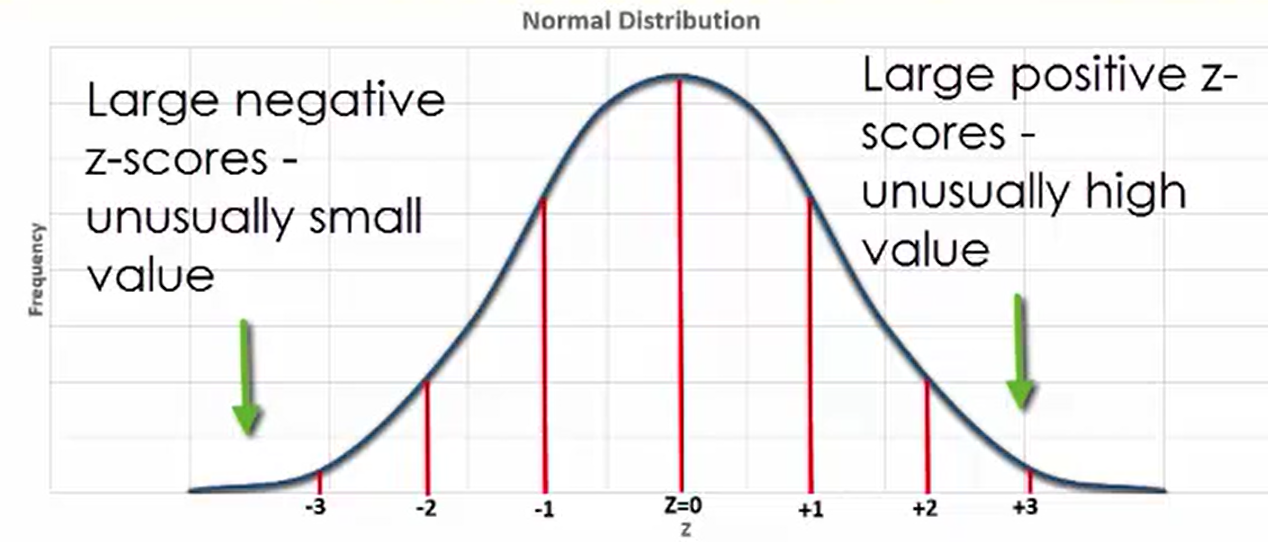When looking at a normally distributed variable, approximately 68% of observations will fall within one standard deviation of the mean, being either greater than or less than the mean value.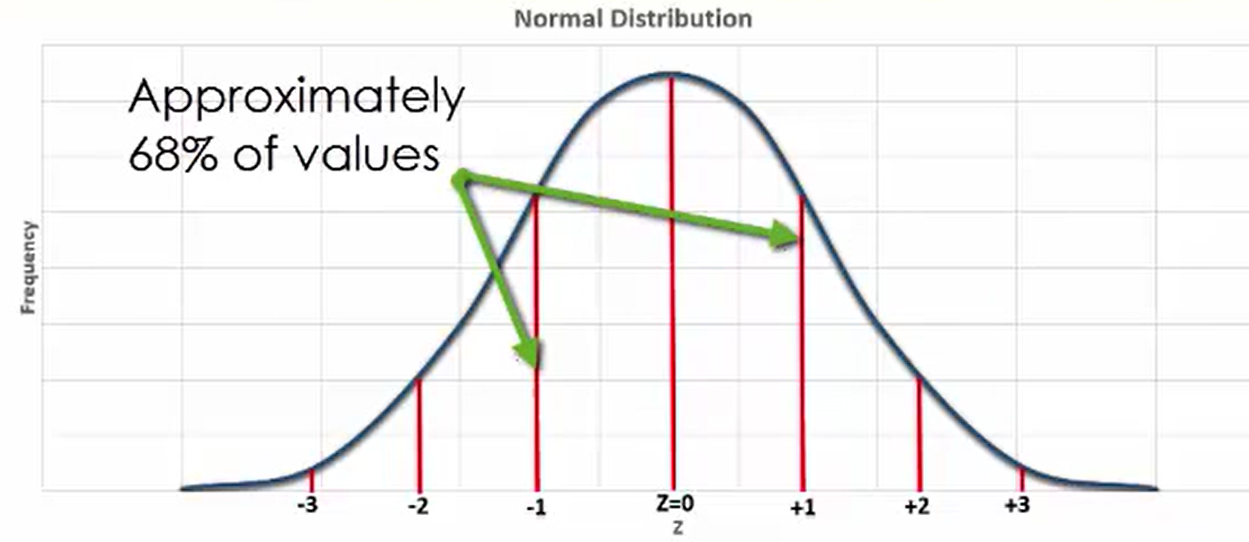Additionally, 95% of observations will fall within two standard deviations of the mean, and 99.7% of observations will fall within three standard deviations of the mean.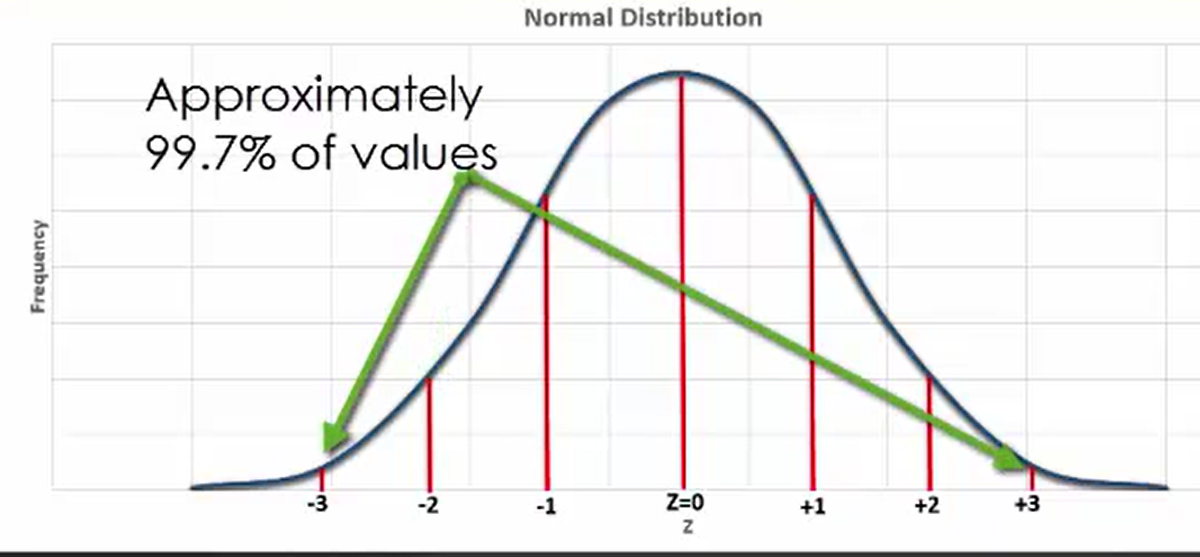This being the case, 95% of sample means will have a z-score that falls between -2 and 2. Sample means in this range indicate that they are likely to occur within a particular sample.

## 2. Determining the Probability of a Mean

When you know the z-score of a sample mean, you can determine the probability of its occurrence by looking up an area corresponding to the specific z-score in a z-table.

z-Table: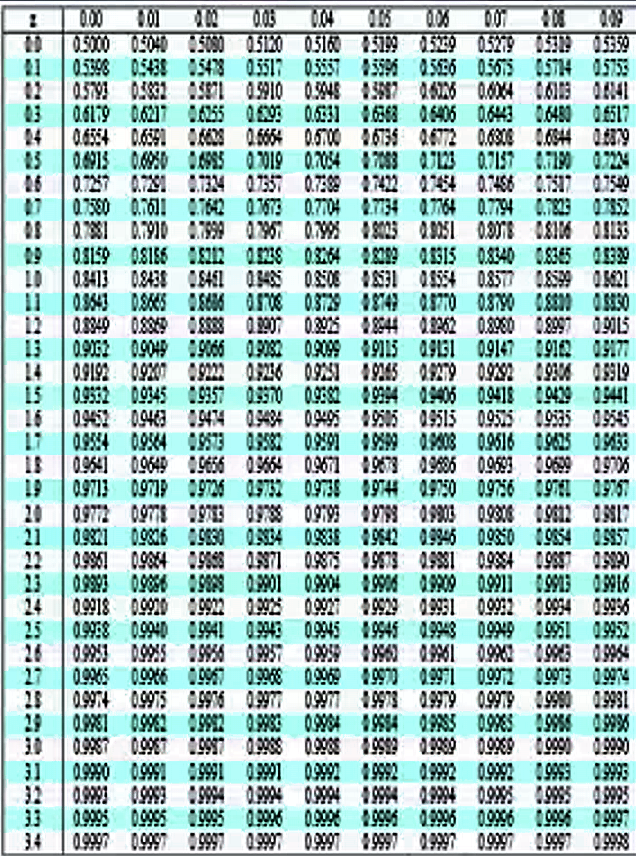The area, which extends from the z-score moving away from the mean, is called a one-sided tail area.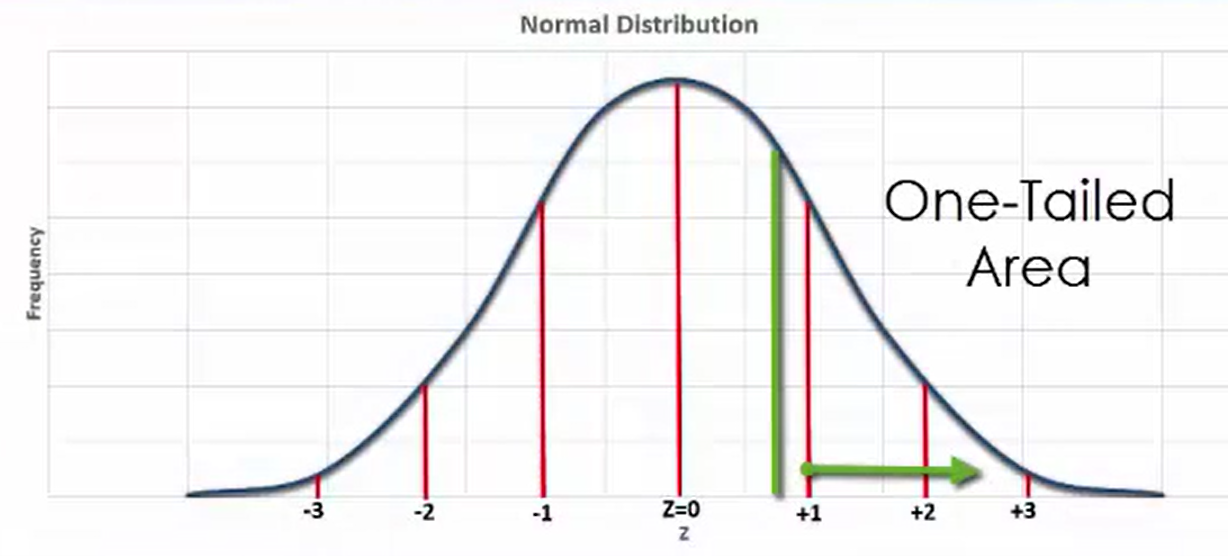If you double this one-sided tail area, you get the two-sided tail area.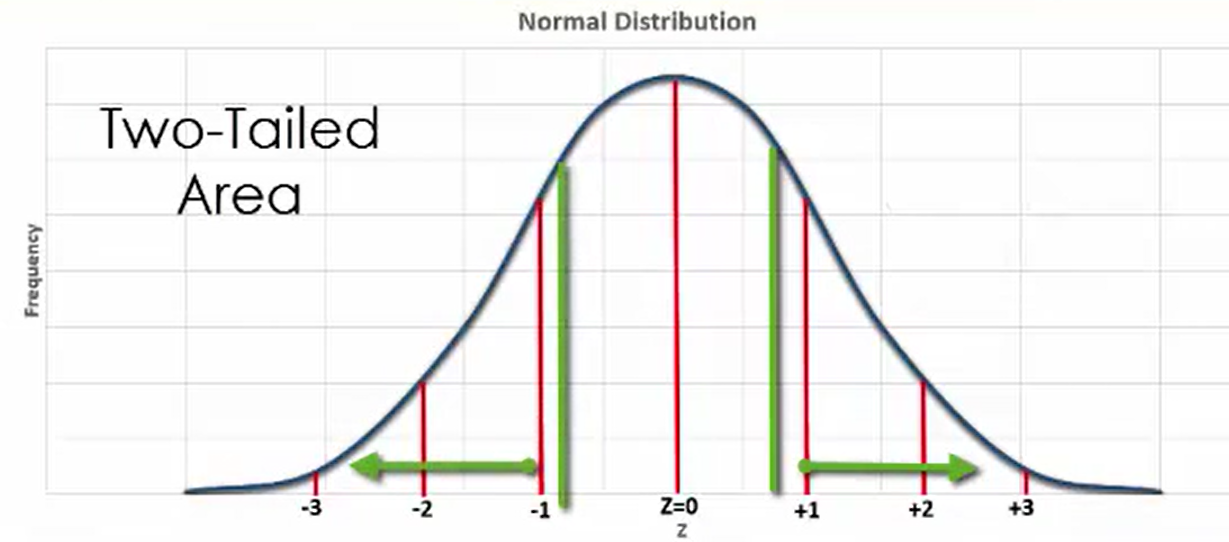Consider the number of friends you may have on Facebook. If the population mean is 175 and the standard deviation is 40, the large majority of observations, roughly 95% of them, will fall between the range of z-scores of -2 and 2, or between 95 and 255 friends.

In this distribution, it would be improbable that the number of Facebook friends of a randomly chosen person would be a very high number, such as 500. It would also be quite improbable that it would be very low, such as 20. On the other hand, some very probable numbers of friends for a randomly selected person would be 150 or 220; in other words, values that fall within a couple of standard deviations of the mean number of 175.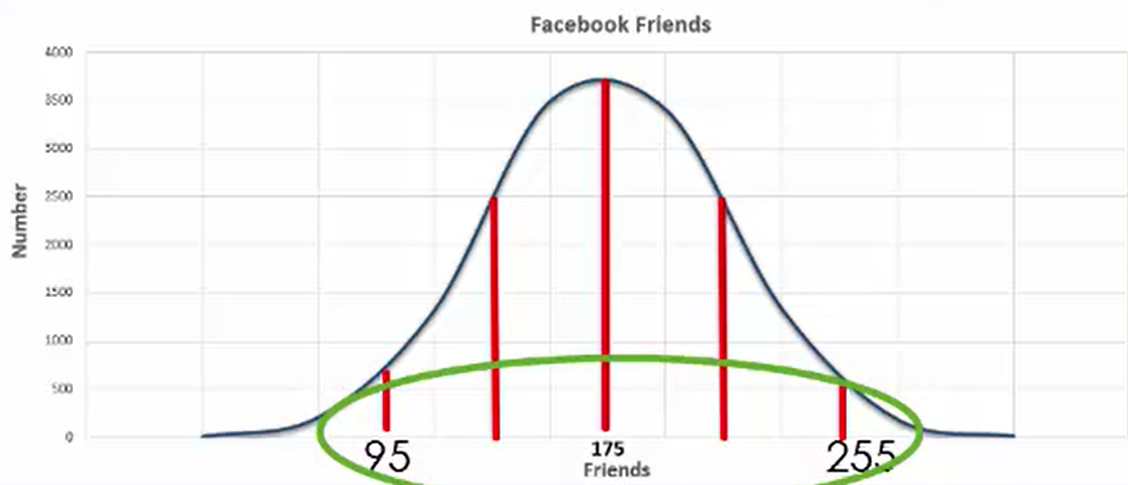Suppose that you were interested in the distribution of women’s shoe sizes, and knew that the mean of the population was a size 8.4, and a population standard deviation was 1.3. How can you determine the probability of the sample mean being equal to a particular shoe size?

First, the normal distribution should be changed to a standard normal distribution by using the z-score equation: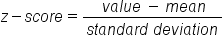Next, assume that the sample mean is a size 8.21 with a sample standard deviation of 0.13 and a sample size of 100. With this, the z-score would be equal to 8.21 minus 8.4 and the difference divided by 0.13, which is approximately -1.46.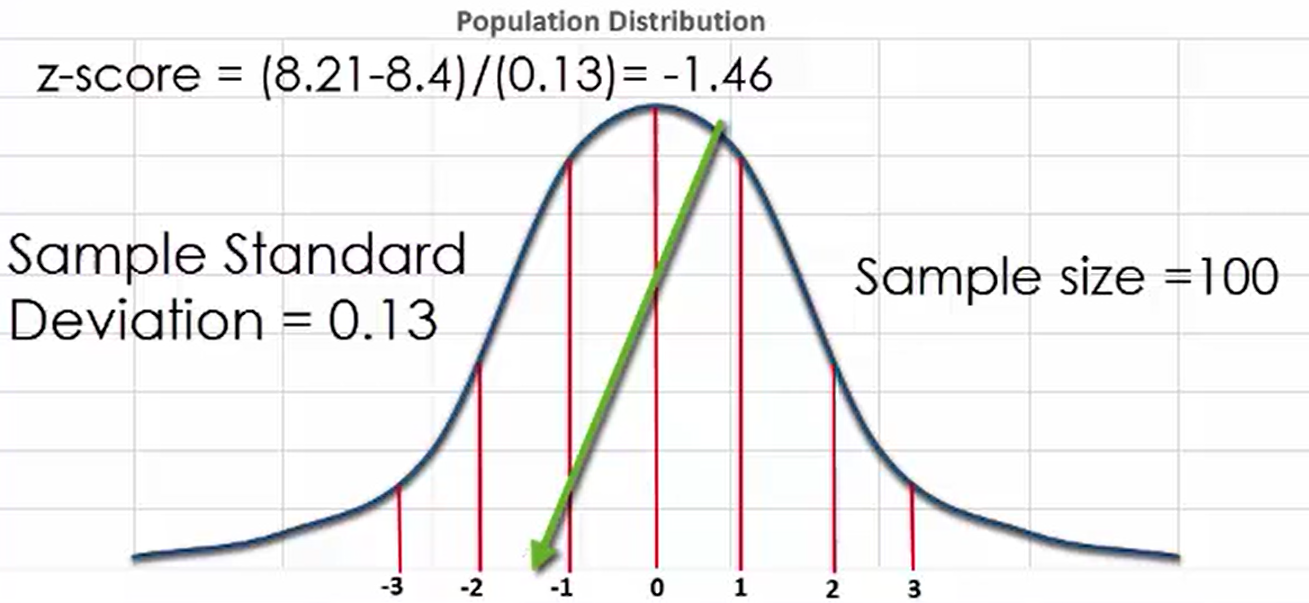try it
What if the mean is 8.54 instead? What is the the z-score?

The z-score would be equal to 8.54 minus 8.4 divided by 0.13, or approximately 1.08.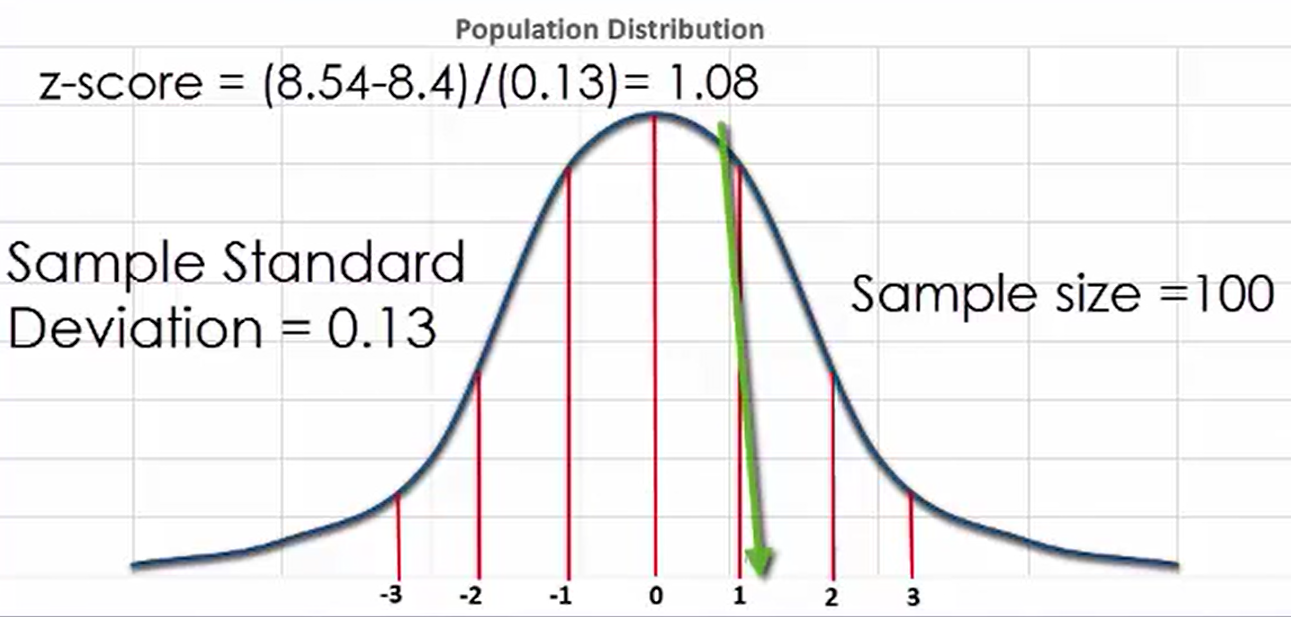What if the mean is size 8.45? What is the z-score?

The z-score would be 8.45 minus 8.4 divided by 0.13. The z-score would be equal to approximately 0.38.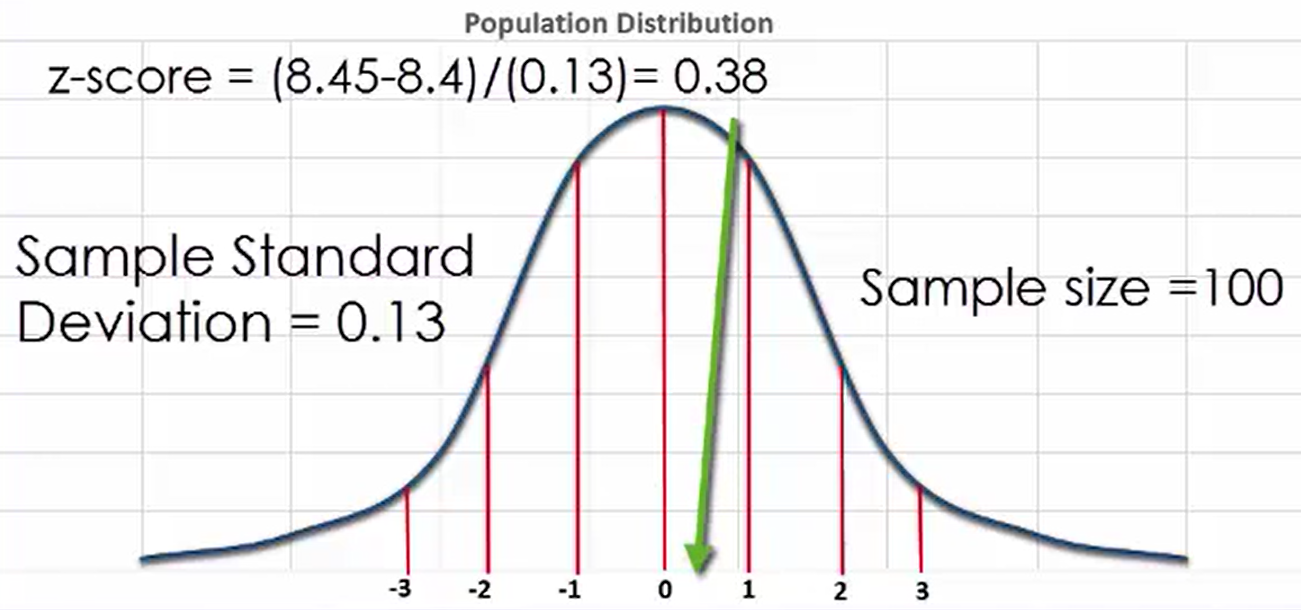Is a 0.38 score probable or improbable?

Since this is less than one standard deviation above the population mean, it is very probable that a sample mean could be equal to 8.45.

Under the graph, you see the one-sided tail area. When you double this, you see the two-sided tail area. Since these areas are quite large, there is a strong probability that the sample mean of less than 8.45 would occur. Large areas such as this indicate a high probability of the mean being less than the selected value. In this case, the z-score of 0.38 translates to a probability of approximately 0.65, but the sample mean would be less than or equal to 8.45.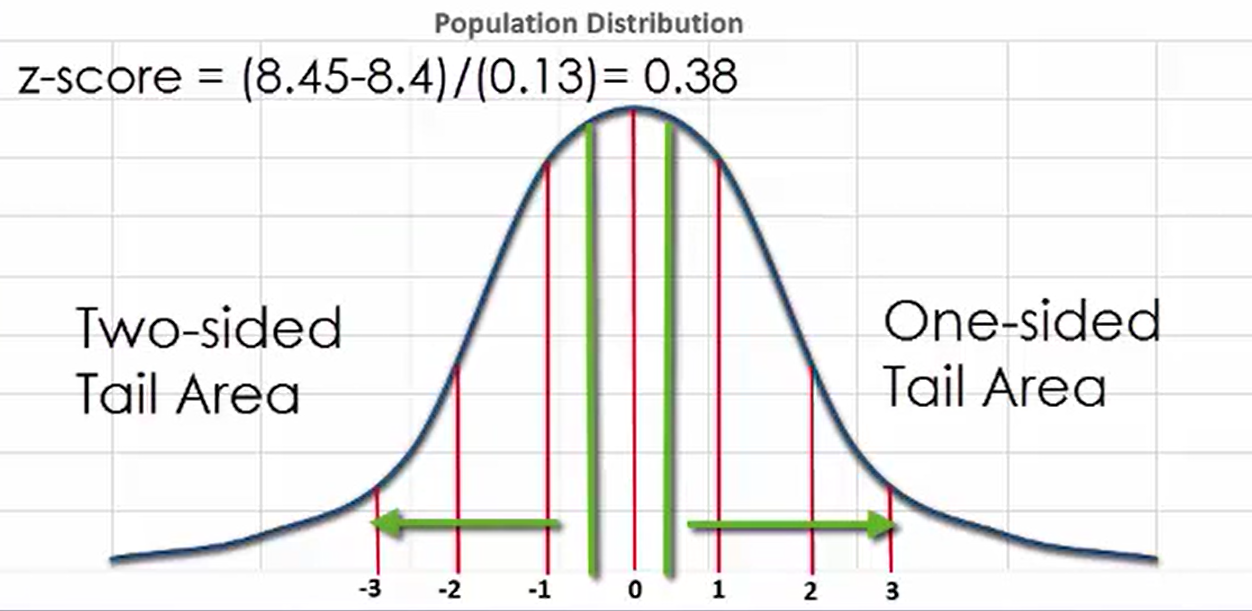Consider an unusually high value of a size 8.8 and see how probable a sample mean size greater than that value is to occur. As you can see in the graph, this value falls on the far right side of the distribution. The z-score for this value is determined as follows: 8.8 minus 8.4 divided by 0.13, which is approximately equal to 3.08.

This corresponds to a one-tailed area of 1 minus 0.999 or approximately 0.001. If we double the area to calculate the two-tailed area, you arrive at a probability of approximately 0.002. This is a very small area and therefore indicates that a mean this large would be very improbable. In general, small areas mean that there is a low probability of a value occurring.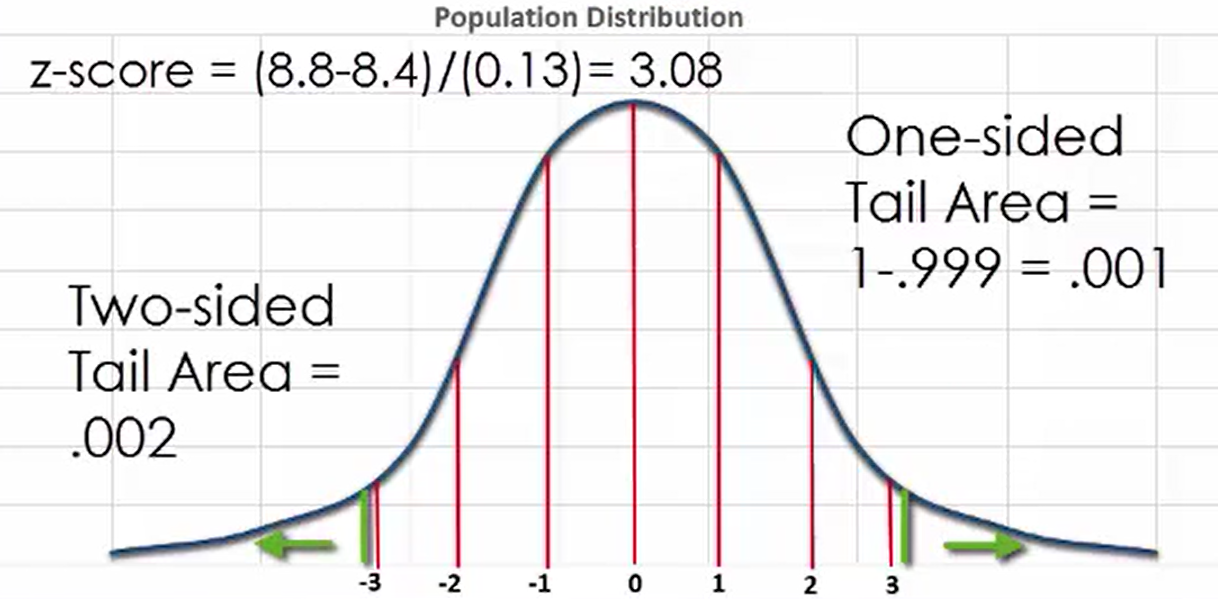term to know
z-Table
Table for looking up the area starting at z = 0 for a positive z-score
summary
This lesson looked at random sampling and how you can use the sampling distribution in determining the probability of a mean occurring. Means with a small two-tailed area are not likely to occur, while means with a large two-tailed area are very probable.

Source: This work is adapted from Sophia author Dan Laub.

Terms to Know
Z-Table

Table for looking up the area starting at z=0 for a positive z-score.

Rating